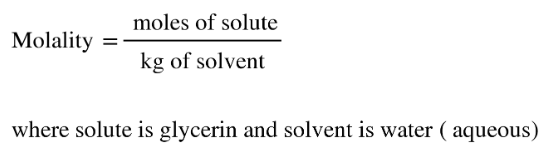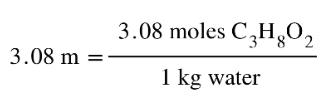# Problem: How could you prepare a 3.08 m aqueous solution of glycerin, C3H8O3? What is the freezing point of this solution?

🤓 Based on our data, we think this question is relevant for Professor Zysmilich's class at GWU.

###### FREE Expert Solution
• Recall that molality can be calculated as:• Therefore, if we have 3.08 m of glycerin, this will appear as:• We need to calculate for the mass of C3H8Ousing the provided moles and MM. Assuming that we will be using 1 kg of solvent/water###### Problem Details

How could you prepare a 3.08 m aqueous solution of glycerin, C3H8O3? What is the freezing point of this solution?